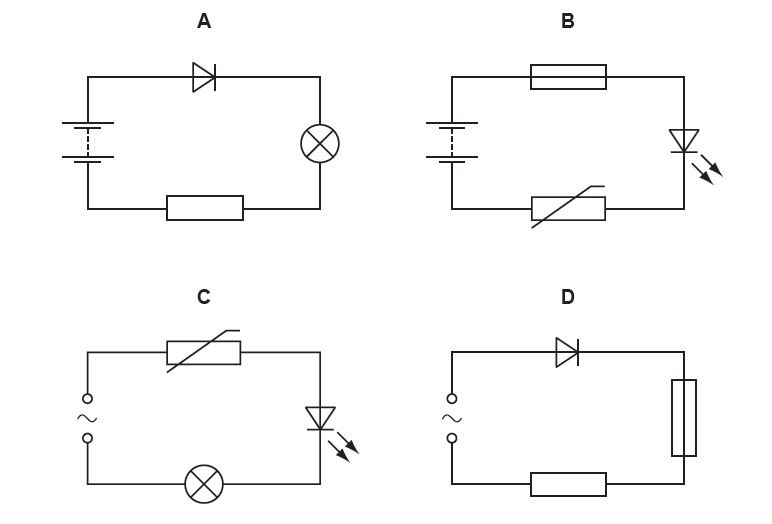## 2018 WAEC GCE Physics Answers for Objective & Essay Questions Released.

The Waec gce physics 2018 answers are detailed below. The West African Examination Council (WAEC) Physics paper for GCE will be taken on Thursday, 13th September, 2018.

The WAEC GCE Physics 2 (Essay) paper will start by 9:30am and will last for 1hr 30mins while the physics 1 (Objective) exam will commence 11am and will last for 1hr 15mins.

In this post, we will be posting out samples of the waec gce physics questions for candidates that will participate in the examination from past questions.### WAEC Physics Obj Questions 2018:

Section A [Objective]

1. An electron moves with a speed of 2.00 x 107 ms-1 in an orbit in a uniform magnetic field of 1.20 x 10-3T.  Calculate the radius of the orbit.
[ Mass of an electron  =  9.11 x 10-31kg; charge on an electron  =  1.61 x 10-19C]

2. A tennis ball projected at an angle attains a range R = 78 m. If the velocity imparted to the ball by the racket is 30ms-1 , calculate .
[g = 10ms-2]

3. A metallic bar 50 cm long has a uniform cross-sectional area of 4.0 cm2.  If a tensile force of 35kN produces an extension of 0.25 mm, calculate the value of Young’s modulus.

4. A stone projected horizontally from the top of a tower with a speed of 4 ms-1 lands on the level ground at a horizontal distance of 25 m from the foot of the tower. Calculate the height of the tower.
[ g = 10 ms-2 ]

5. A spiral spring with a metal extends by 10.5cm in air When the metal Is fully in
water, the spring extends bv 6.8 cm. Calculate the relative density of the metal (Assume Hooke s law Is obeyed)

6. A ray of light is incident on an air-glass boundary at an angle t? If the angle between the partial reflected ray and the refracted ray is 90°, calculate θ given that the refractive index of glass is 1.50.

7. A ball is projected horizontally from a height with a velocity of 40 ms-1. Calculate the drop in height after travelling a horizontal distance of 30m.
[Neglect air resistance; g = 10 ms-1]